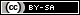# MathsFaculty

A place for maths teachers, just like you, to share original resources.

## Scaffolded trigonometry worksheets#### Resource Type: Worked examples, Faded worked examples, Worksheet - Print

Scaffolded worksheets where students practice finding unknown sides and angles using SOHCAHTOA. Students are guided to label sides, choose a ratio, write their equation, solve the equation and give the answer. There are three separate sheets: one where the unknown side is the numerator, one where the unknown side is the denominator and one where the unknown is the angle.

12 August 2022 Edit: 12 August 2022

Shared by

This resource has been shared under a Creative Commons license:
Attribution-Share AlikeClick thumbnails for selected previews.

PDF